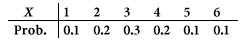### CFA Practice Question

There are 434 practice questions for this study session.

### CFA Practice Question

Suppose we have a loaded die that gives the outcomes 1 through 6 according to the following probability distribution.Note that for this die all outcomes are not equally likely, as they would be if this were a fair die. If this die is rolled 6000 times, the number of times we get a 2 or a 3 should be about ______.

A. 1000
B. 2000
C. 3000